International
Tables for
Crystallography
Volume B
Reciprocal space
Edited by U. Shmueli

International Tables for Crystallography (2006). Vol. B, ch. 3.4, pp. 389-390   | 1 | 2 |

## Section 3.4.8. Derivation of the accelerated convergence formula via the Patterson function

D. E. Williamsa

aDepartment of Chemistry, University of Louisville, Louisville, Kentucky 40292, USA

### 3.4.8. Derivation of the accelerated convergence formula via the Patterson function

| top | pdf |

The structure factor with generalized coefficientsis defined by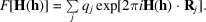The corresponding Patterson function is defined by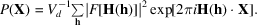The physical interpretation of the Patterson function is that it is nonzero only at the intersite vector points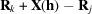. If the origin point is removed, the lattice sum may be expressed as an integral over the Patterson function. This origin point in the Patterson function corresponds to intersite vectors withand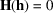: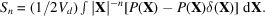Using the incomplete gamma function as a convergence function, this formula expands into two integrals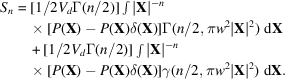The first integral is shown only for a consistent representation; actually it will be reconverted to a sum and evaluated in direct space. The first part of the second integral will be evaluated with Parseval's theorem and the second part in the limit as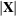approaches zero: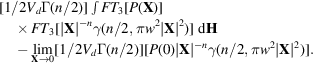The first Fourier transform (of the Patterson function) is the set of amplitudes of the structure factors and the second Fourier transform has already been discussed above; the method for obtaining the limit (for n equal to or greater than 1) was also discussed above. The result obtained is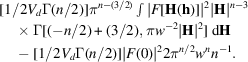The integral can be converted into a sum, since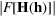is nonzero only at the reciprocal-lattice points: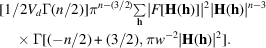The term withis evaluated in the limit, for n greater than 3, as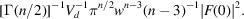Since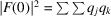, this term is identical with the third term of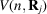as derived earlier. The case ofis handled in the same way as previously discussed, where the limit of this term is zero provided the unit cell has no net charge or dipole moment.